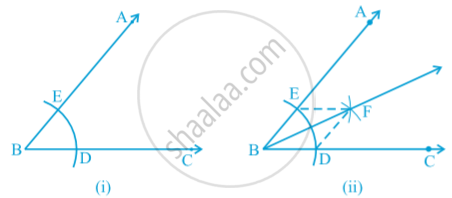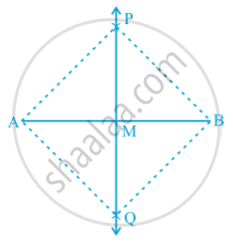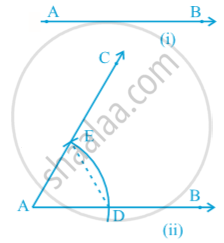# Basic Constructions

#### text

Construction: To construct the bisector of a given angle.
Given an angle ABC, we want to construct its bisector.
Steps of Construction :
1. Taking B as centre and any radius, draw an arc to intersect the rays BA and BC, say at E and D respectively [see fig.(i)]
2. Next, taking D and E as centres and with the radius more than 1/2 DE, draw arcs to intersect each other, say at F.
3. Draw the ray BF [see Fig.(ii)]. This ray BF is the required bisector of the angle ABC.Let us see how this method gives us the required angle bisector.
Join DF and EF.
In triangles BEF and BDF,
BE = BD (Radii of the same arc)
EF = DF (Arcs of equal radii)
BF = BF (Common)
Therefore, ∆BEF ≅ ∆BDF (SSS rule)
This gives ∠EBF = ∠ DBF (CPCT)

Construction:  To construct the perpendicular bisector of a given line segment.
Given a line segment AB, we want to construct its perpendicular bisector.
Steps of Construction :
1. Taking A and B as centres and radius more than 1/2 AB,  draw arcs on both sides of the line segment AB (to intersect each other).
2. Let these arcs intersect each other at P and Q. Join PQ see following fig.3. Let PQ intersect AB at the point M. Then line PMQ is the required perpendicular bisector of AB.
Let us see how this method gives us the perpendicular bisector of AB.
Join A and B to both P and Q to form AP, AQ, BP and BQ.
In triangles PAQ and PBQ,
AP = BP (Arcs of equal radii)
AQ = BQ (Arcs of equal radii)
PQ = PQ (Common)
Therefore, ∆ PAQ ≅ ∆ PBQ (SSS rule)
So, ∠ APM = ∠ BPM (CPCT)
Now in triangles PMA and PMB,
AP = BP (As before)
PM = PM (Common)
∠ APM = ∠ BPM (Proved above)
Therefore, ∆ PMA ≅ ∆ PMB (SAS rule)
So, AM = BM and ∠ PMA = ∠ PMB (CPCT)
As ∠ PMA + ∠ PMB = 180° (Linear pair axiom),
we get
∠ PMA = ∠ PMB = 90°.
Therefore, PM, that is, PMQ is the perpendicular bisector of AB.

Construction:   To construct an angle of 600 at the initial point of a given ray.
Let us take a ray AB with initial point A (see following fig.(i)) We want to construct a ray AC such that ∠ CAB = 60°. One way of doing so is given below.Steps of Construction :
1. Taking A as centre and some radius, draw an arc of a circle, which intersects AB, say at a point D.
2. Taking D as centre and with the same radius as before, draw an arc intersecting the previously drawn arc, say at a point E.
3. Draw the ray AC passing through E [see Fig (ii)].
Then ∠ CAB is the required angle of 60°. Now, let us see how this method gives us the required angle of 60°.
Join DE.
Then, AE = AD = DE (By construction)
Therefore, ∆ EAD is an equilateral  triangle  and the  ∠ EAD,  which is the same as ∠ CAB is equal to 60°.

If you would like to contribute notes or other learning material, please submit them using the button below.

### Shaalaa.com

Basic Constructions part 1 [00:20:05]
S
0%